Best TensorFlow Books 2022 to Build Machine Learning Models

TensorFlow is the most popular library for working with machine learning. It is equally useful for individuals and businesses ranging from startups to companies as large as Google. TensorFlow is currently being used for natural language processing, artificial intelligence, computer vision, and predictive analytics.

If you want to master this technology, reading good books is crucial. Today in this article, I've gathered a number of best TensorFlow books 2022 using a machine learning algorithm as well. So that you can be assured to choose them without any concern. So hurry up and scroll down the list!

1. Hands-On Machine Learning with Scikit-Learn, Keras, and TensorFlow: Concepts, Tools, and Techniques to Build Intelligent Systems

Hands-On Machine Learning with Scikit-Learn and TensorFlow is a very good book to start your journey with machine learning. If you don't know much about deep learning, don't worry.

This practical book shows you how you can use simple and efficient tools to implement programs that are capable of learning from data. With simple and concrete examples, minimal theory and two production-ready Python frameworks, it helps you to understand the basic concepts and tools for building an intelligent system.

To make this book worth reading, you just need a little programming experience.

What You will learn:

• Fundamental machine learning concepts

• Basics of neural network

• Using scikit-learn to track an example machine learning project

• Working with support vector machine and decision trees

• Using random forests and ensemble methods

• Using the TensorFlow library to train neural nets

• Creating neural network architectures, including convolutional nets, recurrent nets, and deep reinforcement learning

• Useful techniques for training and scaling deep neural networks

2. Learning TensorFlow.js: Powerful Machine Learning in JavaScript

You'll begin by working through some basic examples in TensorFlow.js before diving deeper into neural network architectures, DataFrames, TensorFlow Hub, model conversion, transfer learning, and more. Once you finish this book, you'll know how to build and deploy production-readydeep learning systems with TensorFlow.js.

• Explore tensors, the most fundamental structure of machine learning
• Convert data into tensors and back with a real-world example
• Combine AI with the web using TensorFlow.js
• Use resources to convert, train, and manage machine learning data
• Build and train your own training models from scratch

3. Learning Deep Learning: Theory and Practice of Neural Networks, Computer Vision, Natural Language Processing, and Transformers Using TensorFlow

Throughout, Ekman provides concise, well-annotated code examples using TensorFlow with Keras. Corresponding PyTorch examples are provided online, and the book thereby covers the two dominating Python libraries for DL used in industry and academia. He concludes with an introduction to neural architecture search (NAS), exploring important ethical issues and providing resources for further learning.

• Explore and master core concepts: perceptrons, gradient-based learning, sigmoid neurons, and back propagation
• See how DL frameworks make it easier to develop more complicated and useful neural networks
• Discover how convolutional neural networks (CNNs) revolutionize image classification and analysis
• Apply recurrent neural networks (RNNs) and long short-term memory (LSTM) to text and other variable-length sequences
• Master NLP with sequence-to-sequence networks and the Transformer architecture
• Build applications for natural language translation and image captioning

4. Building Machine Learning Pipelines: Automating Model Life Cycles with TensorFlow

Data scientists, machine learning engineers, and DevOps engineers will discover how to go beyond model development to successfully productize their data science projects, while managers will better understand the role they play in helping to accelerate these projects.

• Understand the steps that make up a machine learning pipeline
• Build your pipeline using components from TensorFlow Extended
• Orchestrate your machine learning pipeline with Apache Beam, Apache Airflow and Kubeflow Pipelines
• Work with data using TensorFlow Data Validation and TensorFlow Transform
• Analyze a model in detail using TensorFlow Model Analysis
• Examine fairness and bias in your model performance
• Deploy models with TensorFlow Serving or convert them to TensorFlow Lite for mobile devices
• Understand privacy-preserving machine learning techniques

5. The TensorFlow Workshop: A hands-on guide to building deep learning models from scratch using real-world datasets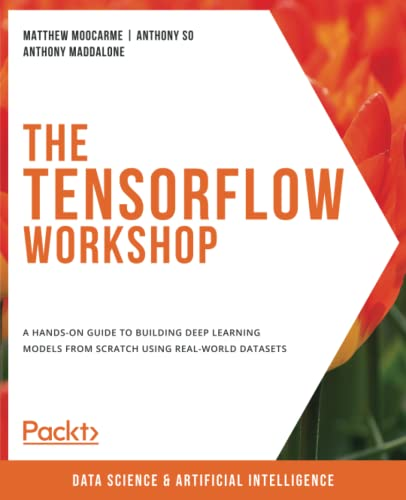Author: Moocarme, Matthew
Published at: 15/12/2021
ISBN: 1800205252

You'll start off with the basics – learning how to load data into TensorFlow, perform tensor operations, and utilize common optimizers and activation functions. As you progress, you'll experiment with different TensorFlow development tools, including TensorBoard, TensorFlow Hub, and Google Colab, before moving on to solve regression and classification problems with sequential models. Building on this solid foundation, you'll learn how to tune models and work with different types of neural network, getting hands-on with real-world deep learning applications such as text encoding, temperature forecasting, image augmentation, and audio processing.

What you will learn

• Get to grips with TensorFlow's mathematical operations
• Pre-process a wide variety of tabular, sequential, and image data
• Understand the purpose and usage of different deep learning layers
• Perform hyperparameter-tuning to prevent overfitting of training data
• Use pre-trained models to speed up the development of learning models
• Generate new data based on existing patterns using generative models

6. Advanced Natural Language Processing with TensorFlow 2: Build effective real-world NLP applications using NER, RNNs, seq2seq models, Transformers, and more

The book covers key emerging areas such as generating text for use in sentence completion and text summarization, bridging images and text by generating captions for images, and managing dialogue aspects of chatbots. You will learn how to apply transfer learning and fine-tuning using TensorFlow 2. Further, it covers practical techniques that can simplify the labelling of textual data. The book also has a working code that is adaptable to your use cases for each tech piece.

What you will learn

• Grasp important pre-steps in building NLP applications like POS tagging
• Use transfer and weakly supervised learning using libraries like Snorkel
• Do sentiment analysis using BERT
• Apply encoder-decoder NN architectures and beam search for summarizing texts
• Use Transformer models with attention to bring images and text together
• Build apps that generate captions and answer questions about images using custom Transformers
• Use advanced TensorFlow techniques like learning rate annealing, custom layers, and custom loss functions to build the latest DeepNLP models

7. TinyML: Machine Learning with TensorFlow Lite on Arduino and Ultra-Low-Power Microcontrollers

Pete Warden and Daniel Situnayake explain how you can train models small enough to fit into any environment. Ideal for software and hardware developers who want to build embedded systems using machine learning, this guide walks you through creating a series of TinyML projects, step-by-step. No machine learning or microcontroller experience is necessary.

• Build a speech recognizer, a camera that detects people, and a magic wand that responds to gestures
• Work with Arduino and ultra-low-power microcontrollers
• Learn the essentials of ML and how to train your own models
• Train models to understand audio, image, and accelerometer data
• Explore TensorFlow Lite for Microcontrollers, Google’s toolkit for TinyML
• Debug applications and provide safeguards for privacy and security
• Optimize latency, energy usage, and model and binary size

8. Python Machine Learning By Example: Build intelligent systems using Python, TensorFlow 2, PyTorch, and scikit-learn, 3rd Edition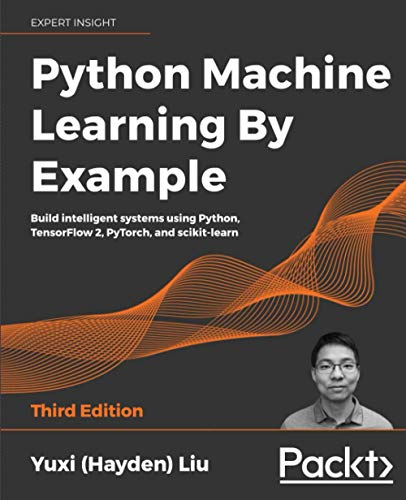Author: Liu, Yuxi (Hayden)
Published at: 30/10/2020
ISBN: 1800209711

With six new chapters, on topics including movie recommendation engine development with Naive Bayes, recognizing faces with support vector machine, predicting stock prices with artificial neural networks, categorizing images of clothing with convolutional neural networks, predicting with sequences using recurring neural networks, and leveraging reinforcement learning for making decisions, the book has been considerably updated for the latest enterprise requirements. This book provides actionable insights on the key fundamentals of ML with Python programming. Hayden applies his expertise to demonstrate implementations of algorithms in Python, both from scratch and with libraries.

Each chapter walks through an industry-adopted application. With the help of realistic examples, you will gain an understanding of the mechanics of ML techniques in areas such as exploratory data analysis, feature engineering, classification, regression, clustering, and NLP.

What you will learn

• Understand the important concepts in ML and data science
• Use Python to explore the world of data mining and analytics
• Scale up model training using varied data complexities with Apache Spark
• Delve deep into text analysis and NLP using Python libraries such NLTK and Gensim
• Select and build an ML model and evaluate and optimize its performance
• Implement ML algorithms from scratch in Python, TensorFlow 2, PyTorch, and scikit-learn

9. TensorFlow Machine Learning Cookbook: Over 60 recipes to build intelligent machine learning systems with the power of Python, 2nd Edition

This is a quick starter guide to learn how to work with the TensorFlow library effectively. It follows a recipe-based approach that practically explains all the concepts of machine learning and TensorFlow library.

This book will teach how you can use TensorFlow for complex data computations and help you gain more insights into your data than ever before.

It also provides advanced techniques that bring more accuracy and speed to machine learning.

What You Will Learn:

• Basics of the TensorFlow machine learning library including variables, matrices, and various data sources

• Exploring Linear Regression techniques with TensorFlow

• Working with Support Vector Machines

• Building  training models

• model evaluation

• Applying sentiment analysis, regression analysis, and clustering analysis

• Building neural networks to improve predictions

• Natural Language Processing

• Building Convolutional Neural Networks

• Taking TensorFlow to Production

10. TensorFlow for Machine Intelligence: A Hands-On Introduction to Learning Algorithms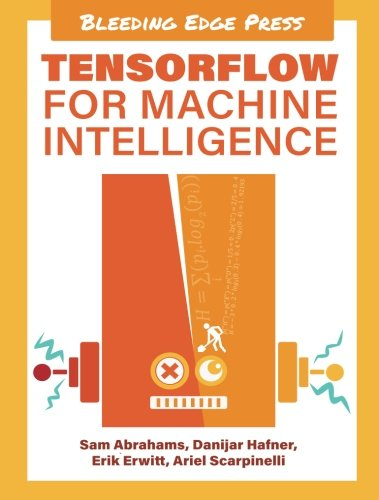Author: Sam Abrahams,Danijar Hafner,Erik Erwitt,Ariel Scarpinelli
Published at: 10/11/2016
ISBN: 1939902452

This book gives a proper overview of TensorFlow in a clear and concise manner. It's suitable for anyone from beginner to the advanced level machine learning practitioner.

By using concrete and real-world examples, it introduces you to the TensorFlow framework and the underlying machine learning concepts that are important to work with machine intelligence.

Completing this book, you'll have a deep understanding of the core TensorFlow API.

What You Will learn:

• Introduction to deep learning and TensorFlow library

• Basics of machine learning

• Object recognition and classification with convolutional neural networks

• Building recurrent neural networks

• Natural language processing

• Deploying models in production

• Writing helper function

• Code structure and classes

11. Learning TensorFlow: A Guide to Building Deep Learning Systems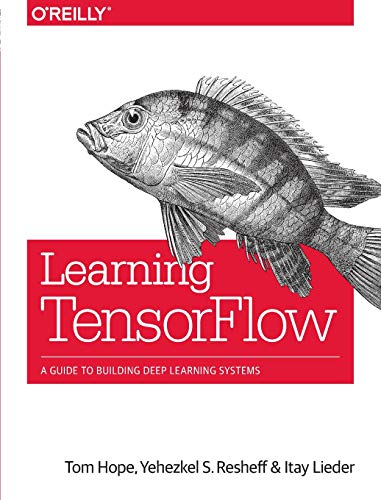Author: Tom Hope,Yehezkel S. Resheff,Itay Lieder
Published at: 27/08/2017
ISBN: 1491978511

Learning TensorFlow is a hands-on guide that will teach you all the aspects of Tensorflow--from basics to advanced level concepts.

It begins with some basic examples in TensorFlow and gradually dives deeper into topics such as neural network architecture, TensorBoard visualization, and multithreaded input pipelines. With this book, you'll be able to build and deploy production-ready deep learning systems in TensorFlow.

It's suitable for a broad technical audience—from data scientists and engineers to students and researchers.

What You Will learn:

• Understanding TensorFlow fundamentals

• Using TensorFlow to build deep learning models from scratch

• Convolutional neural networks

• Working with text and sequence using TensorBoard visualization

• Word vectors, advanced RNN, and embedding visualization

• Using TensorFlow abstraction to make  development easier and faster

• Using clusters to distribute model training

• Exporting and serving models

12. Pro Deep Learning with TensorFlow: A Mathematical Approach to Advanced Artificial Intelligence in Python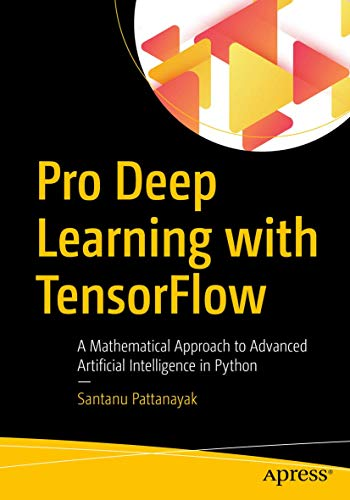Author: Santanu Pattanayak
Published at: 07/12/2017
ISBN: 1484230957

This TensorFlow book is ideal for anyone who wants to become an expert in deep learning. Using the popular deep learning library, TensorFlow, it provides practical expertise to make you understand how to build and deploy meaningful deep learning solutions from scratch.

With this book, you'll be able to use TensorFlow to optimize different deep learning architectures. You can build a new deep learning application using the prototypes demonstrated in the book. You'll also develop the mathematical understanding and intuition required to invent new deep learning architectures and solutions on your own.

What You Will learn:

• Understanding full stack deep learning using TensorFlow

• Deploying complex deep learning solutions

• Working with different deep learning architectures

• A solid mathematical foundation to understand the concepts better

• Required skills to carry out research on deep learning

• Performing experiments using TensorFlow

13. TensorFlow for Deep Learning: From Linear Regression to Reinforcement Learning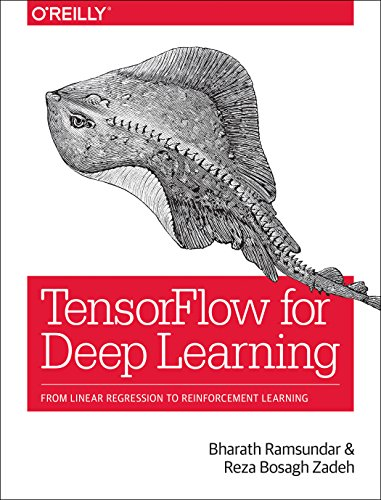Published at: 23/03/2018
ISBN: 1491980451

If you want to understand deep learning from the ground up and build working deep learning models from scratch, then read this book.

This practical book shows you how to and where to use deep learning architectures. With this book, you'll be able to design and build systems capable of detecting objects in images, understanding human speech, analyzing video and predicting the properties of potential medicines.

What You Will Learn:

• Fundamentals of deep learning and TensorFlow

• Working with TensorFlow API and primitives

• Linear regression with TensorFlow

• Building a fully connected Deep network with TensorFlow

• Building convolutional neural networks

• Training and tuning machine learning systems with TensorFlow on large datasets

• Distributed training and reinforcement learning

14. R Deep Learning Cookbook: Solve complex neural net problems with TensorFlow, H2O and MXNet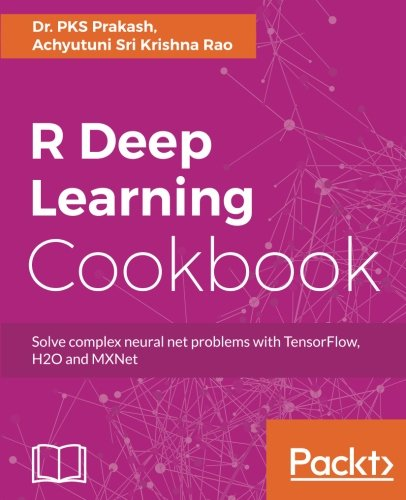Author: Dr. PKS Prakash,Achyutuni Sri Krishna Rao
Published at: 04/08/2017
ISBN: 1787121089

R Deep Learning Cookbook provides powerful and independent recipes to build deep learning models in different application areas using R and TensorFlow libraries.

With practical and working examples to build systems for text, image and speech recognition. You'll also be able to set-up deep learning models using CPU and GPU.

What You Will learn:

• Building deep learning models using TensorFlow, H2O, and MXnet

• Analyzing a Deep Boltzmann machine

• Building a supervised model using various machine learning algorithms

• Setting up and Analysing Deep belief networks

• Representing data using Autoencoders

• Building sequence modeling using Recurrent nets

•  Applying Deep Learning in text mining

•  Using generative models available in Deep Learning

• Understanding basic convolution function

• Fundamentals of Reinforcement Learning

• Applying deep learning in signal processing

• Training a deep learning model on a GPU

15. TensorFlow 1.x Deep Learning Cookbook: Over 90 unique recipes to solve artificial-intelligence driven problems with Python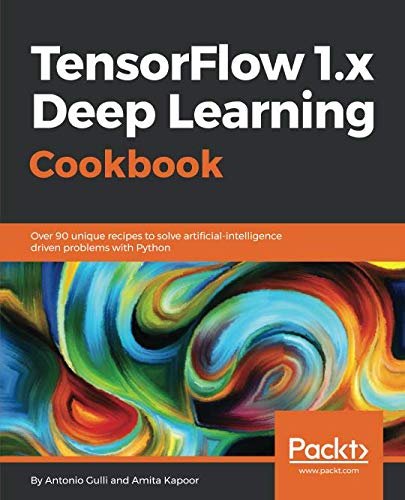Author: Antonio Gulli,Amita Kapoor
Published at: 12/12/2017
ISBN: 1788293592

If you want to develop your skill to implement advanced techniques in deep learning using TensorFlow, then try this book.

This practical book full of real-world examples will teach you how to apply deep learning techniques in various applications. You will understand how to implement different deep neural architectures in Tensorflow.

You'll also learn how to use TensorFlow in different mobile and embedded platforms and set up cloud platforms for deep learning applications.

What You Will learn:

• Installing and using TensorFlow for CPU and GPU options

• Implementing Deep neural networks

• Using the TensorBoard to understand the architecture of the neural network

• Using different regression techniques for the task of prediction and classification

• Implementing convolutional neural networks

• Building recurrent neural networks to perform the task of text generation

• Understanding the implementation of Autoencoders, and deep belief networks

16. Mastering TensorFlow 1.x: Advanced machine learning and deep learning concepts using TensorFlow 1.x and Keras

Mastering TensorFlow 1.x is a comprehensive guide that helps you to master all the core and advanced machine learning concepts using the TensorFlow book.

Covering basic uses of TensorFlow library, including TensorFlow Core, TensorBoard, and TensorFlow Serving it'll take you through machine learning algorithms from Classification, Regression, and Clustering, and many more domains.

Completing this book, you will be able to build and scale neural network models and deep learning applications of your own.

What You Will Learn:

• Advanced concepts of Tensorflow 1.x like Ensembles, Transfer Learning, Reinforcement Learning and GAN

• Performing unsupervised learning (Clustering) with TensorFlow

• Performing supervised learning (Classification, Regression) with TensorFlow

• Building end-to-end Deep Learning (CNN, RNN, Autoencoders) models with TensorFlow

• Working with varied datasets such as MNIST, CIFAR-10, and the latest YouTube-8M database

• Building TensorFlow models with Keras, TFLearn, and R

• Scaling and deploying production models with distributed and high-performance Tensorflow on GPU and Clusters

• Building TensorFlow models on iOS and Android devices

17. Machine Learning with TensorFlow

Machine Learning with TensorFlow will teach you machine learning algorithms and how to implement solutions with TensorFlow.

It starts with an overview of machine learning concepts and moves on to the essentials needed to begin using TensorFlow. Each chapter zooms into a prominent example of machine learning.

You can cover them all to master the basics.

What You Will Learn:

• Fundamental machine learning concepts

• Basics of TensorFlow

• Building neural networks

• Solving various classification and prediction problems in the real world

• Regression analysis

• Building machine learning models to solve various classification and prediction problems in the real world

• Using cluster for distributed model training

18. Deep Learning with TensorFlow 2 and Keras: Regression, ConvNets, GANs, RNNs, NLP, and more with TensorFlow 2 and the Keras API, 2nd Edition

You'll learn how to write deep learning applications in the most powerful, popular, and scalable machine learning stack available. TensorFlow is the machine learning library of choice for professional applications, while Keras offers a simple and powerful Python API for accessing TensorFlow. TensorFlow 2 provides full Keras integration, making advanced machine learning easier and more convenient than ever before. This book also introduces neural networks with TensorFlow, runs through the main applications (regression, ConvNets (CNNs), GANs, RNNs, NLP), covers two working example apps, and then dives into TF in production, TF mobile, and using TensorFlow with AutoML.

What you will learn

• Build machine learning and deep learning systems with TensorFlow 2 and the Keras API
• Use Regression analysis, the most popular approach to machine learning
• Understand ConvNets (convolutional neural networks) and how they are essential for deep learning systems such as image classifiers
• Use GANs (generative adversarial networks) to create new data that fits with existing patterns
• Discover RNNs (recurrent neural networks) that can process sequences of input intelligently, using one part of a sequence to correctly interpret another
• Apply deep learning to natural human language and interpret natural language texts to produce an appropriate response
• Train your models on the cloud and put TF to work in real environments
• Explore how Google tools can automate simple ML workflows without the need for complex modeling

Thanks for reading this post. If you have any opinion don't hesitate to comment here. Also please subscribe our newsletter to get more updates.